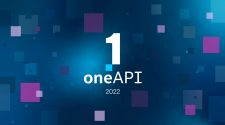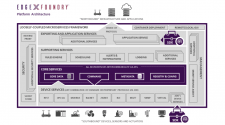In this tutorial, you’ll be introduced to 6 handy Python data visualization libraries, and learn how to choose among them.

Data visualization is a great way to analyze and present information; Python is one of the most popular data analysis languages. This results in many good Python libraries for data visualizations. There is no single best Python data visualization tool, so it could be hard to pick the right one to use.

In this guide, you’ll learn about the below popular Python data visualization libraries:

• `Matplotlib`
• `Seaborn`
• `Plotly (Dash)`
• `Bokeh`
• `Folium`
• `plotnine`

If you want to start using Python for data visualization, this article is a great starting point. With an overview of the top libraries, including an example chart with code, you’ll have a much better idea to choose among them.

Let’s get started!

## Before we start

To use Python for data visualization, you’ll need to know its basics. You can learn with the resources below:

This list of Python data visualization libraries starts with the more commonly used ones, to the ones that satisfy a specific need. We’ll introduce each of the libraries with:

• an overview
• an example chart with code
• a summary

For comparisons purpose, we’ll plot the same chart for all the applicable libraries. The code is also attached for you to get a sense of using such a library.

Below is the code to generate the dataset. Don’t worry about the details. All you need to know is that at the end, we have a `pandas` DataFrame called `tips`. Each row of this dataset represents a restaurant bill. We’ll only use its two columns `total_bill` and `tip` to show the example scatter plot. For details about this dataset, check out the document.

 from plotly import data tips = data.tips() type(tips) # pandas.core.frame.DataFrame
view rawimport_data.py hosted withby GitHub

## 1. Matplotlib

`<a href="https://matplotlib.org/" target="_blank" rel="noreferrer noopener">Matplotlib</a>` is the most well-known foundation data visualization library in Python. It’s widely used by data science beginners, to experts in the field. If you’ve used MATLAB before, you’ll find `matplotlib` has some similarities.

It is a comprehensive library for creating static, animated, and interactive visualizations in Python. With `matplotlib`, you can:

• build various 1D/2D charts, almost all you can think of! Including line charts, bar charts, histograms, heatmaps, box plots, scatter plots, contour, and so on.
• also make 3D plotting with its extension toolkit `mplot3d`.
• almost take full control of the customization of plots, including line styles, font, and axes.

But because `matplotlib` is highly customizable, it is complicated and not intuitive to learn and use within a short time. As a result, many third-party packages extend and build on `matplotlib` functionality. For example, `pandas`, the powerful data analysis and manipulation library in Python, offers basic plotting functions based on `matplotlib`. Also, `seaborn`, a higher-level library covered in this article, is built on `matplotlib`.

 import matplotlib.pyplot as plt plt.plot(tips[‘total_bill’], tips[‘tip’], ‘o’) plt.xlabel(‘total_bill’) plt.ylabel(‘tip’) plt.show()
view rawmatplotlib.py hosted withby GitHub
 tips.plot(x=‘total_bill’, y=‘tip’, kind=‘scatter’) # pandas function
view rawpandas.py hosted withby GitHub

Summaryfoundation and comprehensive library, highly customizable, not very easy to learn and use

## 2. Seaborn

`Seaborn` is a Python library for making statistical data visualization. As mentioned earlier, it is a higher-level library that’s based on `matplotlib`. It is also integrated closely with pandas data structures, which makes it easy to learn and use.

With `seaborn`, you can focus on the meaning of different elements of the charts, rather than on the details of how to plot them. With its functions’ default settings, you can generate nice-looking and informative plots. While you still have options to customize the charts further to fit your need. But, when you need very particular customization, you still need `matplotlib` as a compliment, if `seaborn` doesn’t offer that in its functions.

`Seaborn` is especially useful for exploring and understanding data. Some of the functionalities that `seaborn` offers:

• options for visualizing univariate and bivariate distributions.
• options for visualizing numerical and categorical variables.
• automatic estimation and plotting of linear regression model fit.
• ability to build complex visualizations such as multi-plot grids.

Please compare the below example to the `matplotlib` example. `Seaborn` has much less code than `matplotlib` to get the same stylish chart.

 import seaborn as sns sns.relplot(data=tips, x=‘total_bill’, y=‘tip’)
view rawseaborn.py hosted withby GitHub

Summaryeasy to learn and use, good for exploratory data analysis, relatively customizable, can integrate with matplotlib if need more customizations

Unlock the power of `seaborn `by exploring an example dataset with histograms, heatmaps, scatter plots, barplots, etc.

## 3. Plotly (Dash)

`<a href="https://plotly.com/python/" target="_blank" rel="noreferrer noopener">Plotly</a>` is also a popular Python data visualization library.

Its recommended starting point of the `<a href="https://plotly.com/python/plotly-express/" target="_blank" rel="noreferrer noopener">Plotly Express</a> module` offers convenient functions like `seaborn` for creating the most common figures. Then, we can also add customizations to it with other functions. Besides popular 1D/2D charts like line plots, scatter plots, histograms, maps, `Plotly` also offers 3D visualization functions.

The characteristic that makes `Plotly` standing out is we can create interactive graphs. We can interact with the plots by zooming in and out, and so on, which makes them easier to explore and better to present.

Besides `Plotly`, the same company also offers another package called `Dash`. We can use it to build interactive web applications. When using `Dash`, we can use `Plotly `to create the data visualizations within the dashboard.

 import plotly.express as px px.scatter(tips, x=‘total_bill’, y=‘tip’)
view rawplotly.py hosted withby GitHub

Summaryeasy to use and customizable, create interactive graphs and web applications

Plotly Python Tutorial: How to create interactive graphs
Learn how to create a basic figure quickly with `Plotly Express`, then add customization to it.
6 Steps to Interactive Python Dashboards with Plotly Dash
Learn how to create Python interactive dashboards with `Plotly Dash` with an example.

## 4. Bokeh

Similar to `Plotly (Dash)``Bokeh` also helps us create interactive plots or web applications.

Both `Bokeh` and `Plotly Dash` have their advantages and disadvantages. But, we prefer `Dash` since it is easier to learn, and offers stylish and more plots than `Bokeh`. With that said, `Bokeh` was founded earlier and has a higher volume of downloads. So it is a great option as well.

 from bokeh.plotting import figure, output_notebook, show output_notebook() p = figure() p.circle(x=tips[‘total_bill’], y=tips[‘tip’]) p.xaxis.axis_label = “total_bill” p.yaxis.axis_label = “tip” show(p)
view rawbokeh.py hosted withby GitHub

Summarycreate interactive graphs and web applications

## 5. Folium

`Folium` is a Python tool for making map visualizations. We can manipulate data in Python, then visualize it on an interactive leaflet map. We can plot choropleth visualizations as well as passing data as markers on the map.

Other Python libraries we’ve mentioned earlier also offer map plots, but none of them is dedicated like `folium`.

 import folium m = folium.Map(location=[40.730610, –73.935242], zoom_start=12) folium.Marker( [40.706005, –74.008827], tooltip=‘Wall Street’ ).add_to(m) folium.Marker( [40.748817, –73.985428], tooltip=‘Empire State Building’ ).add_to(m) folium.Marker( [40.689247, –74.044502], tooltip=‘Statue of Liberty’ ).add_to(m) m
view rawfolium.py hosted withby GitHub

Summarygood for nice looking map graphs

## 6. plotnine

If you’ve used R before, you probably have tried the` ggplot2` library. There’s a Python library called `plotnine` based on `ggplot2` in R`plotnine` also implements the so-called grammar of graphics. The grammar makes it easy for us to compose plots by explicitly mapping data to the visual objects that make up the plot. We can also make a variety of charts with `plotnine`.

This library has a pretty different syntax than other popular Python data visualization libraries. But if you are familiar with R, or you like the mindset of using ‘grammar’ to build plots, `plotnine` is a good option.

 from plotnine import ggplot, geom_point, aes ggplot(tips, aes(‘total_bill’, ‘tip’)) + geom_point(color=‘blue’)
view rawggplot.py hosted withby GitHub

Summarygrammar of graphics, based on the R `ggplot2 `library

That’s it!

In this post, you’ve learned about 6 Python data visualization libraries.

Below is a summary of the general guide to choose among them:

• Explore and understand data: `seaborn`
• Create highly customized charts: `matplotlib`
• Present data as interactive graphs or web dashboards: `Plotly (Dash)` or `Bokeh`
• Generate nice-looking maps: `folium`
• Stick to a similar style as R `ggplot2` in Python: `plotnine`

There are other helpful Python data visualization libraries, but this list should cover the most common usage.

Hope now you know which data visualization library to use!

We’d love to hear from you. Leave a comment for any questions you may have or anything else.

This feature was originally sourced from JustIntoData.

### Suggested Posts##### IBM Bolsters Investments in CEE, Extends Client Engineering In Prague, Warsaw And Moscow##### Bio Farma Collaborates with Google Cloud and ‘ConnectedLife With Fitbit’ To Empower Healthier Living And Chronic Disease Prevention Across Indonesia##### Cloudflare And Oracle Join Forces To Help Eliminate Data Transfer Fees And Ease Path To Multicloud##### Cloudflare Acquires Vectrix To Help Businesses Gain Visibility And Control Of Their Applications##### Intel Innovation: Developer/oneAPI News